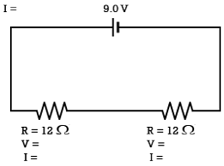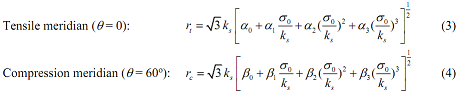+1-415-670-9189
info@expertsmind.com

# Get Solution

Determine a unique set of the constant values
Course:- Mechanical Engineering
Reference No.:- EM132187179

 TweetExpertsmind Rated 4.9 / 5 based on 47215 reviews.
Review Site
Assignment Help >> Mechanical Engineering

Applied Elasticity and Plasticity Project -

Use a computer program to draw the yield surfaces of the yield criteria given in the table below (a) on deviatoric planes at ρ = 0 and four other different values, where ρ = I1/√3 = √3σm = √3σoct; (b) on meridain planes at θ = 0o, 15o, 30o, 45o, and 60o. (c) Also draw the 3-D perspective views of the yield surfaces in the principal-stress space from three different angles.

 Yield Criteria Uniaxial Compressive Yield Stress Uniaxial Tensile Yield Stress von Mises 50,000 psi 50,000 psi Mohr-Coulomb 2,000 psi 200 psi Yield criterion defined below 2,000 psi 200 psi

The yield surface of the yield criterion is expressed in the form:

F(σ) = √3τ0 - r(θ, σ0yc, ks) = 0 (1)

where σ is the stress tensor whose Cartesian components are σij, σ0 = I1/3, I1 = σkk = first invariant of stress tensor, τ0 = √(2J2/3), J2 = sijsij/2 = second invariant of the stress deviator sij = σij - 1/3 δijσkk, δij = Kronecker delta, θ = 1/3 cos-1((3√3)/2)J3J2-3/2) = similarity angle which represents the polar angle in the deviatoric section, J3 = 1/3 sijsjkski = third invariant of sij, σyc is the uniaxial compressive yield stress, ks is a constant which determines the size of the yield surface, and function r(θ, σ0yc, ks) represents the deviatoric section of the yield surface which can be expressed by the formulawhere rt and rc represent the tensile and compression meridians, respectively, which are defined as:The equations above involve eight parameters α0, · · ·, α3, β0,· · · , β3. These eight parameters and constant ks can be determined based on the following conditions:

1. The two apices of each curve of Eq. 3 and Eq. 4 on the horizontal axis must be common to both curves.

2. The point representing the yield stress under uniaxial tension has to be on the yield surface.

3. The point representing the yield stress under uniaxial compression has to be on the yield surface too.

The above conditions are not enough to determine a unique set of the constant values. Use any set of constants fulfill the above conditions for your project. Include a section in your project report to show the values you use and explain and show calculations how you determine the constants.

Note - It has to be done in Matlab. As for the report of 1000 words need Abstract, intro, procedure, discussion of results, conclusion.

Attachment:- Assignment File.rar

View Conversion
Minimizelen2187179 12/6/2018 2:38:26 AM It has to be done in matlab, as for the report of 1000 words i need Abstract, intro, procedure, discussion of results, conclusion. The project is due on the day of the last lecture of this class. Hand in a hardcopy of typed project report by the due date. The report should at least include your thinking and explanation in working out the problem, the computer software you used, how to generate the input data, how to run the program, the computer-generated plots of the yield surfaces, your comments and discussions, and appendixes for the computer printouts of your input data and output results with essential information highlighted.len2187179 12/6/2018 2:38:21 AM Organize your report according to the following general guidelines: Cover page: Giving title and dates, etc. Table of Contents: Listing the sections and figures. Abstract: Summarizing the report including important findings. Introduction: Describing the importance of the work done. Procedure and Results: Including figures and/or tables. Discussion of Results: Giving a thorough analysis and interpretation of results. Conclusions: Giving a summary of the findings. Appendix: Showing important calculations and formulas. Figures and tables should be numbered and must have captions explaining what the figures and tables are illustrating. You must always show units of axes for figures and the units for the numbers listed in the tables.

Ask Question & Get Answers from Experts
Browse some more (Mechanical Engineering) Materials
 Two flow meters, one fitted at the inlet of the heat exchanger for the hot water am the other fitted at that of the cold water. How rate is read by the position of the float At resonance, the amplitude is measured to be 0.8 cm. At 0.85 resonant frequency, the amplitude is measured to be 0.6 cm. Determine the damping factor (zeta) of the system. Air at 300 kPa, 63oC, and a velocity of 423 m/s enters an insulated duct of varying cross-sectional area. The air exits at 150 kPa, 88oC. At the inlet, the cross-sectional are Each of the two arms has weight w per unit length and supports weight W = 10wL at its end. Derive formulas for the maximum shear force and maximum bending moment in the arms An enclosure contains an array of circuit boards, 15 cm high and 20 cm long. The clearance between the tips of the components on the PCB and the back surface of the adjacent P Diffusion of carbon into BCC iron for 12 hours at a temperature of 5000C results in a carbon concentration of 0.2 wt% at a depth 2 mm from the surface. At what depth would the Complete one of the following projects involving methods for storing electricity considered thus far in this book (see Secs. 2.7, 4.8.3). Report your findings in a report pr Determine the view factors F12 and F21 for two concentric spheres characterized by radii R1 and R2, where R2 > R1. What is the view factor when the smaller sphere is displaced